# ISEE Lower Level Math : How to identify the parts of a list

## Example Questions

### Example Question #217 : Data Analysis And Probability

What is the median of the list below?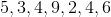Explanation:

The median is the middle number of a set when the numbers are ordered from smallest to largest.

The reorganized version of the set, with the numbers ordered from smallest to largest is: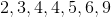Given that the 2 middle numbers are 4, the median is 4. When 2 numbers are both in the middle, the average must be taken. The average of 4 and 4 is 4.

### Example Question #218 : Data Analysis And Probability

If Beth is 12 years old, Bob is 14 years old, Jeremy is 15 years old, and Lisa is 7 years old, what is their mean age?Explanation:

Add up the ages and divide by the number of people: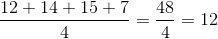Therefore, 12 is the mean.

### Example Question #219 : Data Analysis And Probability

A store is selling bags of apples that are 5 pounds each, but the number of apples per bag varies. The bags contain the following number of appes: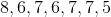What is the mode?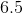Explanation:

The mode is the number that occurs most often in a set.Given that 7 appears three times, more than any other number, it is the mode.

### Example Question #220 : Data Analysis And Probability

On a quiz with only one question, 4 students failed (meaning they got a score of 0), and 5 students passed (meaning they got a score of 1). What is the median score?

None of theseExplanation:

Given that 4 students got a score of 0 and 5 got a score of 1, the scores would look like this when put into a set: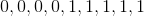The median is the middle number in a sequentially organized set. The middle number in this set is 1, making it the median.

### Example Question #221 : Data Analysis And Probability

What is the missing variable from the set?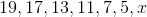Explanation:

To find the value of the missing term, we need to determine the trend in the set.In this case, the set is comprised of prime numbers in decending order. The next term in this series will be 3.

### Example Question #222 : Data Analysis And Probability

What is the mean of the set: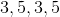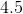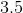Explanation:

The mean of a set is found by dividing the sum of the set by the number of items in the set. This gives us the equation: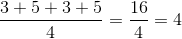The mean is 4.

### Example Question #223 : Data Analysis And Probability

The weight of the apples that Katie bought are given in pounds: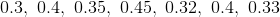What is the median?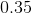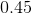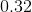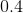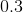Explanation:

The median is the number that is in the middle of the set when ordered smallest to largest.When the set is ordered smallest to largest, the set is: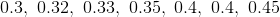Since 0.35 is the fourth number in the set of seven numbers, it is in the middle and therefore the median.

### Example Question #224 : Data Analysis And Probability

Beth runs two miles on Monday, three miles on Tuesday, and two miles on Thursday. What is the mean number of miles that she runs per day?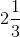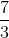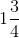Explanation:

The mean is found by taking the sum of the numbers in a set and dividing by the number of items in that set.

Over the four days, Beth runs two miles, three miles, zero miles, and then another two miles, for a sum of seven miles.

Thus, the mean is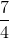, ormiles.

### Example Question #225 : Data Analysis And Probability

5 brothers attend a family reunion. The oldest is 26 years old. If the brothers' ages are spaced by 2 years, how old is the second youngest brother?Explanation:

If the oldest brother is 26 years old, and their ages are all evenly spaced by two years each, then their ages must be as follows: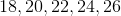The second youngest brother is therefore 20 years old. Thus, 20 is the correct answer.

### Example Question #226 : Data Analysis And Probability

What is the median of the set below?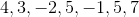Explanation:

The median is the middle number of a set when the numbers are organized from smallest to largest.

In order to find the median of the set,, the first step is to rearrange the numbers from smallest to largest.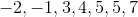Given thatis the middle number of this set, it is the correct answer.